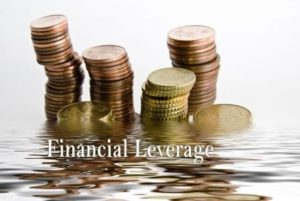# Financial Leverage

## Financial Leverage – Meaning

Financial leverage simply means the presence of debt in the capital structure of a firm. Similarly, in other words, we can also call it the existence of fixed-charge bearing capital which may include preference shares along with debentures, term loans etc. There are basically three leverages; operating leverage, financial leverage, combined leverage. The objective of introducing leverage to the capital is to achieve maximization of wealth of the shareholders.

Financial leverage deals with the profit magnification in general. It is also well known as gearing or ‘trading on equity’. The concept of financial leverage is not just relevant to businesses but it is equally true for individuals. Debt is an integral part of the financial planning of anybody whether it is an individual, firm or a company. We will try to understand it from the business point of view.

In a business, debt (short or long term) is acquired not only on the grounds of ‘need for capital’ but also taken to enlarge the profits accruing to the shareholders. Let me clarify it further. An introduction of debt in the capital structure will not have an impact on the sales, operating profits etc but it will increase the share of the equity shareholders, the ROE % (Return on Equity).## Measures of Financial Leverage

There are various measures of Financial Leverage

• Debt Ratio: It is the ratio of debt to total assets of the firm which means what percentage of total assets is financed by debt.
• Debt Equity Ratio: It is the ratio of debt to equity which signifies how many dollars of debt is taken per dollar of equity.
• Interest Coverage Ratio: It is the ratio of profits to interest. This ratio is also represented in times. It represents how many times of the interest is the available profit to pay it off. Higher such ratio, higher is the interest paying capacity. The reciprocal of it is income gearing.

## Degree of Financial Leverage

A degree of financial leverage is nothing but a measure of magnification that happens due to debt capital in the structure. The degree of financial leverage is the proportion of a percentage change in EPS due to a certain percentage change in EBIT.

 Degree of Financial Leverage (DFL) = % change in EPS % change in EBIT

## Illustration of Financial Leverage

Let’s understand the effect of leverage with the help of the following an example. The calculation below clearly shows the effect of having debt in the capital. The return on equity (ROE) and the EPS both are higher in a case of debt and equity structure.

 Particulars Only Equity Debt – Equity Equity Shares of Rs. 10 Each 5,00,000 2,50,000 Debt @ 12 % 2,50,000 EBIT 1,20,000 1,20,000 Interest 30,000 PBT 1,20,000 90,000 Tax – 50% 60,000 45,000 PAT 60,000 45,000 No. of Shares 50,000 25,000 EPS 1.2 1.8 ROE 12% 18%

The picture shown in the above illustration does not bring all aspects of leverage. Hence, we shall go further inside to know the reason for having higher EPS and ROE in the case of a levered firm. Let us calculate one more important ratio – ROI (Return on Investment). ROI for both the firms will be 24% (EBIT / Total Investment = 120000 / 500000).

Now, here we see that the ROI is more than the interest rate charged by lender i.e. 12%. This is the reason behind the higher EPS as well as ROE in the case of a levered firm. So, leverage would not always be profitable. The following matrix explains the behavior of levering a firm.

 Favorable ROI > Interest rate Unfavorable ROI < Interest Rate Neutral ROI = Interest Rate

In the current example, the first situation i.e. ROI > Interest Rate is true and that is why the results are favorable as we can see. If the ROI is less than the interest rate, the ROE will decline and on the other hand, if ROI is the same as interest rate, it will make no difference.